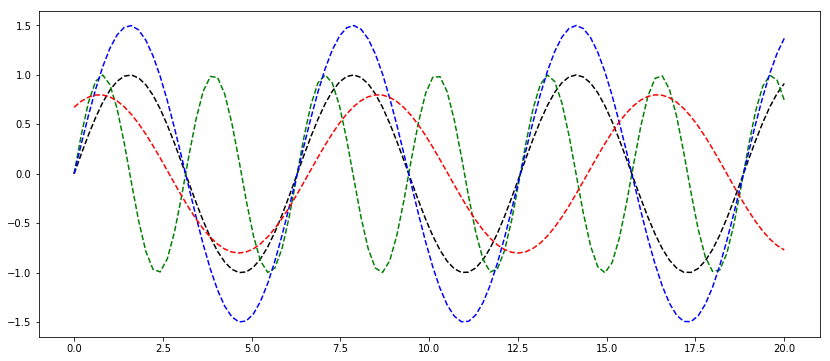# Blogging with Jupyter

by Daniel Pollithy

# Blogging with Jupyter

I realized that most of my blog posts behave like a jupyter notebook (markdown, code and repeat). So why not just write a jupyter notebbok and post it as a blog entry. I am going evaluate if this is as nice as writing with prose.io.

## How it works

1. git clone the repo containing the jekyll blog
2. jupyter notebook run the notebook and write stuff
3. ipython nbconvert <your_notebook>.ipynb --to markdown
4. Move the generated markdown to the “_posts” folder Caution: The images of this notebook are placed into a folder called <your_notebook>_files.
%matplotlib inline

import numpy as np
import matplotlib.pyplot as plt

plt.rcParams['figure.figsize'] = (14, 6)

x = np.linspace(0.0, 20.0, num=100)
y1 = np.sin(x)
y2 = np.sin(x*2)
y3 = 1.5 * np.sin(x)
y4 = 0.8 * np.sin(0.8*x + 1)

plt.plot(x, y1, 'k--', x, y2, 'g--', x, y3, 'b--', x, y4, 'r--')

[<matplotlib.lines.Line2D at 0x7fed42f93710>,
<matplotlib.lines.Line2D at 0x7fed42f937d0>,
<matplotlib.lines.Line2D at 0x7fed42f93fd0>,
<matplotlib.lines.Line2D at 0x7fed42f9d410>]# Tricks with jupyter notebook

• get a plot.ly account and generate interactive graphs
• use %load_ext autoreload %autoreload 2
• display multiple beatiful outputs (not only the last of a cell): from IPython.core.interactiveshell import InteractiveShell InteractiveShell.ast_node_interactivity = "all"
• access the docs by prepending a question mark and evaluating a cell: ?str.replace
• list all ipython magic commands: %lsmagic
• use variables in multiple notebooks like global context: %store data and %store -r data (list all with %who)
• %%time time information of a cell about a single run
• %%timeit runs the same cell 10.000 times and returns the mean of the durance
• run shell commands: !ls -la or !python3 -m install numpy
• LaTeX formulas: \$P(A \mid B) = \frac{P(B \mid A) \, P(A)}{P(B)} \$ $P(A \mid B) = \frac{P(B \mid A) \, P(A)}{P(B)}$

(More ideas: jupyter notebook tips)